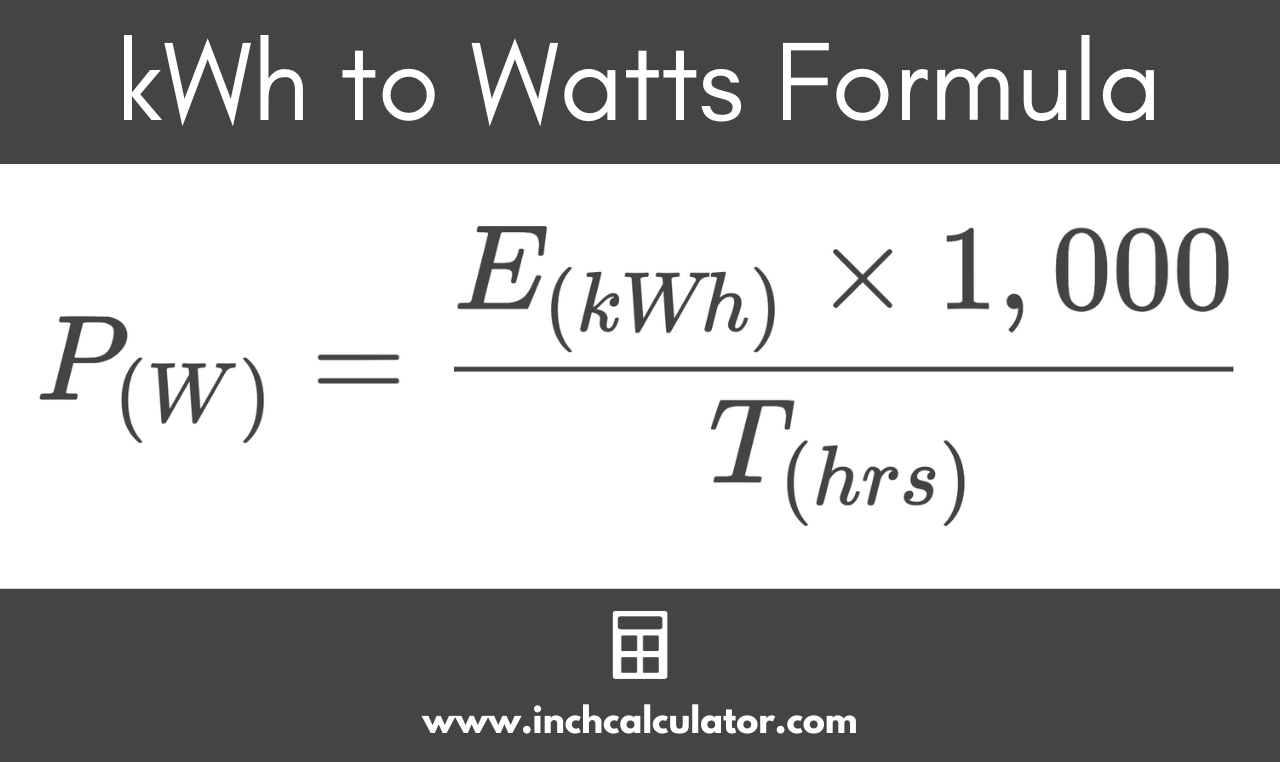# Kilowatt-Hours (kWh) to Watts Conversion Calculator

Convert kilowatt-hours to watts by entering energy in kWh and time in hours.

## Power in Watts:

W
Learn how we calculated this below

## How to Convert Kilowatt-Hours to Watts

A watt (W) is a measure of power, while a kilowatt-hour (kWh) is a measure of energy equivalent to 1,000 watt-hours, or 1,000 watts of power for 1 hour. So, to convert kilowatt-hours to watts, multiply the energy in kilowatt-hours by 1,000 to find the energy in watt-hours, then divide by the time in hours.

### kWh to Watts Formula

Use this formula to convert watts to kilowatt-hours:

P(W) = E(kWh) × 1,000 / T(hrs)

Thus, the power P in watts is equal to the energy E in kilowatt-hours times 1,000, divided by the time T in hours.

For example: let’s find the average watts of power for 3 kWh of energy used in 6.5 hours.

P(W) = 3 kWh × 1,000 / 6.5 hrs
P(W) = 3,000 Wh / 6.5 hrs
P(W) = 461.54 W

So, 3 kilowatt-hours of energy over 6.5 hours will consume 461.54 watts of power.

If you don’t multiply the top half of the fraction by 1,000, then the formula will convert the energy to kilowatts.## Common Kilowatt-Hour to Watt Conversions

Common kilowatt-hour to watt conversions for a 1-hour time period.
Energy in Kilowatt-hours Power in Watts
1 kWh 1,000 W
2 kWh 2,000 W
3 kWh 3,000 W
4 kWh 4,000 W
5 kWh 5,000 W
6 kWh 6,000 W
7 kWh 7,000 W
8 kWh 8,000 W
9 kWh 9,000 W
10 kWh 10,000 W

You might also find our watts to kWh conversion calculator helpful.